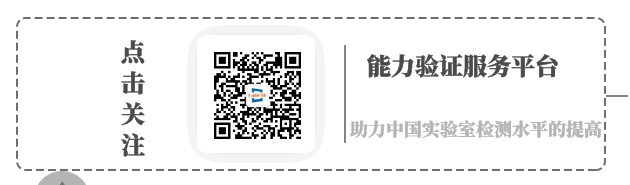0535-2129197

# 能力验证中稳健Z比分数的计算方法

实验室能力验证和评价包括两方面的工作，一方面是计算工作评价；另一方面是作出技术评定。对于不同类型的能力验证方案，所采用的工作评价值计算模型也各不相同。在实际工作中，各实验室的检测比对试验方案主要以求取稳健Z比分数，来评定结果的满意程度；

Z =(х—X)/IQR×0.7413

式中х—参加实验室结果值

X—所有结果值的中位置

IQR—上四分位值与下四分位值之间的差值

判定准则：︱Z ︳≦2 满意结果

2﹤︱Z ︳﹤3 可疑结果

︱Z ︳≧3 不满意结果

式中IQR的计算方法：

计算步骤：

一、确定Q1、Q2、Q3位置Q1位置=（n+1）/4

Q2位置=2（n+1）/4

Q3位置=3（n+1）/4

二、确定相应的四分位数

三、计算标准IQR

IQR=Q3-Q1

标准IQR=0.7413×IQR

例如：各实验室某项检测结果分别是4.7 5.0 6.2 4.0 5.3 4.9 5.7 5.0 4.5

第一步：按顺序排列该组数据

 1 2 3 4 5 6 7 8 9 4 4.5 4.7 4.9 5 5 5.3 5.7 6.2

第二步：确定Q1、Q2、Q3位置

Q1位置=（n+1）/4=（9+1）÷4=2.5

Q2位置=2（n+1）/4=2（9+1）÷4=5

Q3位置=3（n+1）/4=3（9+1）÷4=7.5

Q1=0.5×4.5＋0.5×4.7=4.6
Q2=5.0
Q3=0.5×5.3＋0.5×5.7=5.5

IQR=Q3-Q1=5.5－4.6=0.9
标准IQR（NIQR)=0.7413×IQR=0.7413×0.9=0.667
将IQR带入Z =(х—X)/IQR×0.7413 计算出Z值来判定结果的满意程度。

例如第9个测试值的Z值：Z=（6.2-5）/NIQR=1.79,︱Z ︳≦2 ,结果满意﻿